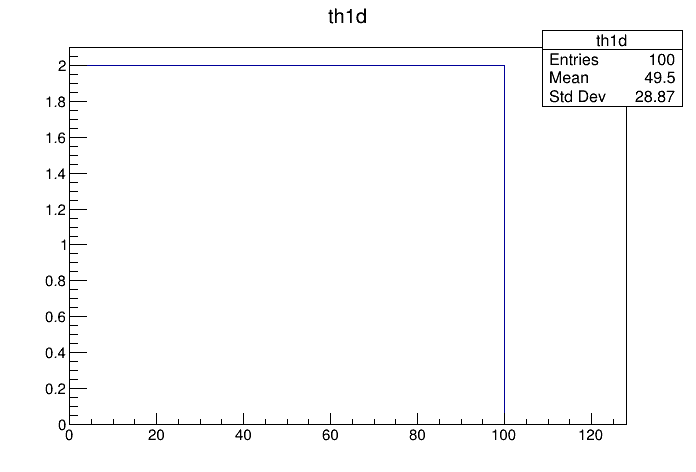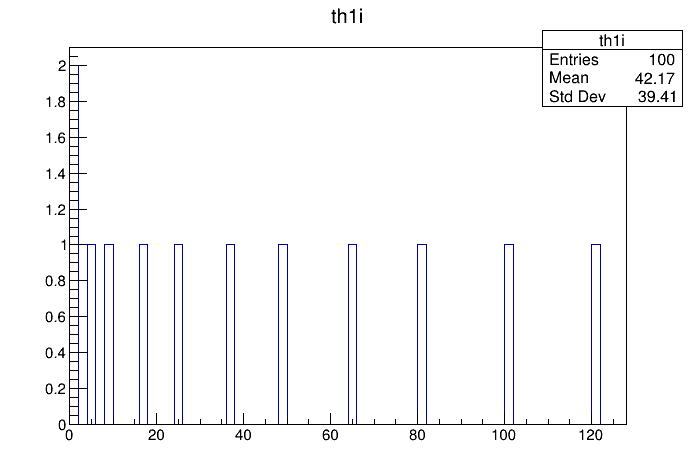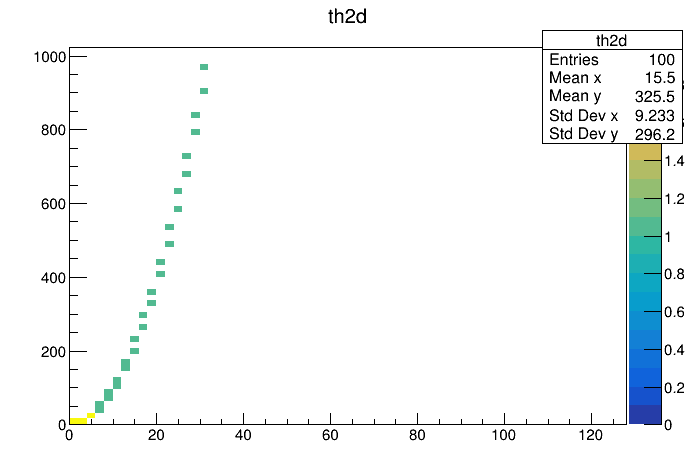# Df 0 0 5_Fill Any Object¶

Using the generic Fill action.

This tutorial shows how to fill any object the class of which exposes a Fill method.

Author: Danilo Piparo (CERN)
This notebook tutorial was automatically generated with ROOTBOOK-izer from the macro found in the ROOT repository on Thursday, June 24, 2021 at 07:11 AM.

A simple helper function to fill a test tree: this makes the example stand-alone.

In :
%%cpp -d
void fill_tree(const char *treeName, const char *fileName)
{
ROOT::RDataFrame d(100);
auto i = 0.;
d.Define("b1", [&i]() { return i; })
.Define("b2",
[&i]() {
float j = i * i;
++i;
return j;
})
.Snapshot(treeName, fileName);
}


We prepare an input tree to run on

In :
auto fileName = "df005_fillAnyObject.root";
auto treeName = "myTree";
fill_tree(treeName, fileName);


We read the tree from the file and create a rdataframe.

In :
ROOT::RDataFrame d(treeName, fileName);


## Filling any object¶

We now fill some objects which are instances of classes which expose a Fill method with some input arguments.

In :
auto th1d = d.Fill<double>(TH1D("th1d", "th1d", 64, 0, 128), {"b1"});
auto th1i = d.Fill<float>(TH1I("th1i", "th1i", 64, 0, 128), {"b2"});
auto th2d = d.Fill<double, float>(TH2D("th2d", "th2d", 64, 0, 128, 64, 0, 1024), {"b1", "b2"});

auto c1 = new TCanvas();
th1d->DrawClone();

auto c2 = new TCanvas();
th1i->DrawClone();

auto c3 = new TCanvas();
th2d->DrawClone("COLZ");

return 0;


Draw all canvases

In :
gROOT->GetListOfCanvases()->Draw()